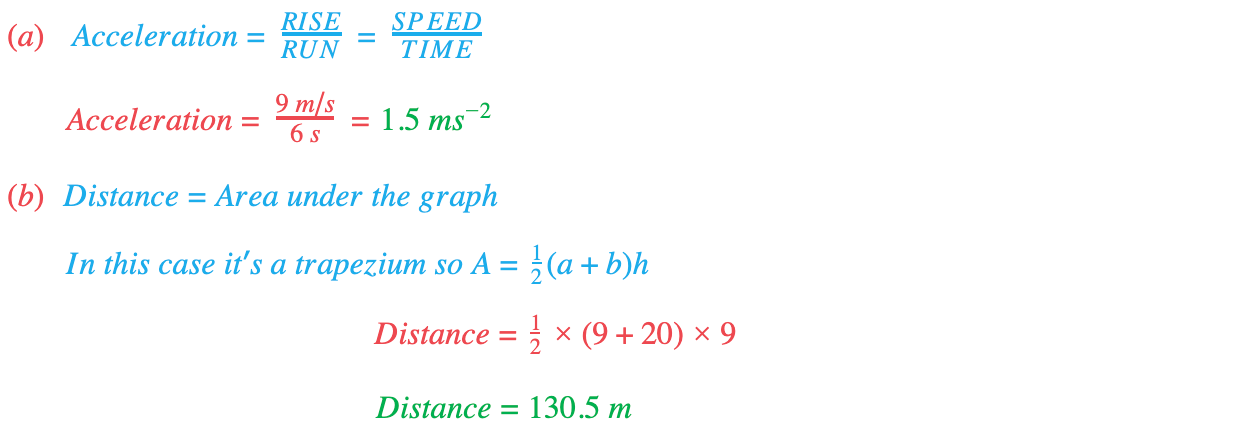# Edexcel IGCSE Maths 复习笔记 3.3.2 Speed-Time Graphs

Edexcel IGCSE Maths 复习笔记 3.3.2 Speed-Time Graphs

#### What is a speed-time graph?

• Speed-time graphs show speed at different times
• Speed is on the vertical axis, and time is on the horizontal axis.

• The gradient of the graph is the acceleration:Acceleration = RISE / RUN = SPEED / TIMEArea under graph = Distance covered

Horizontal line = Constant speed (so zero acceleration)

#### Exam Tip

It is easy to get confused between different types of graph.

Look at the label on the vertical axis to make sure you are looking at a SPEED-time graph (not distance-time).

#### Worked Example#### Worked Example